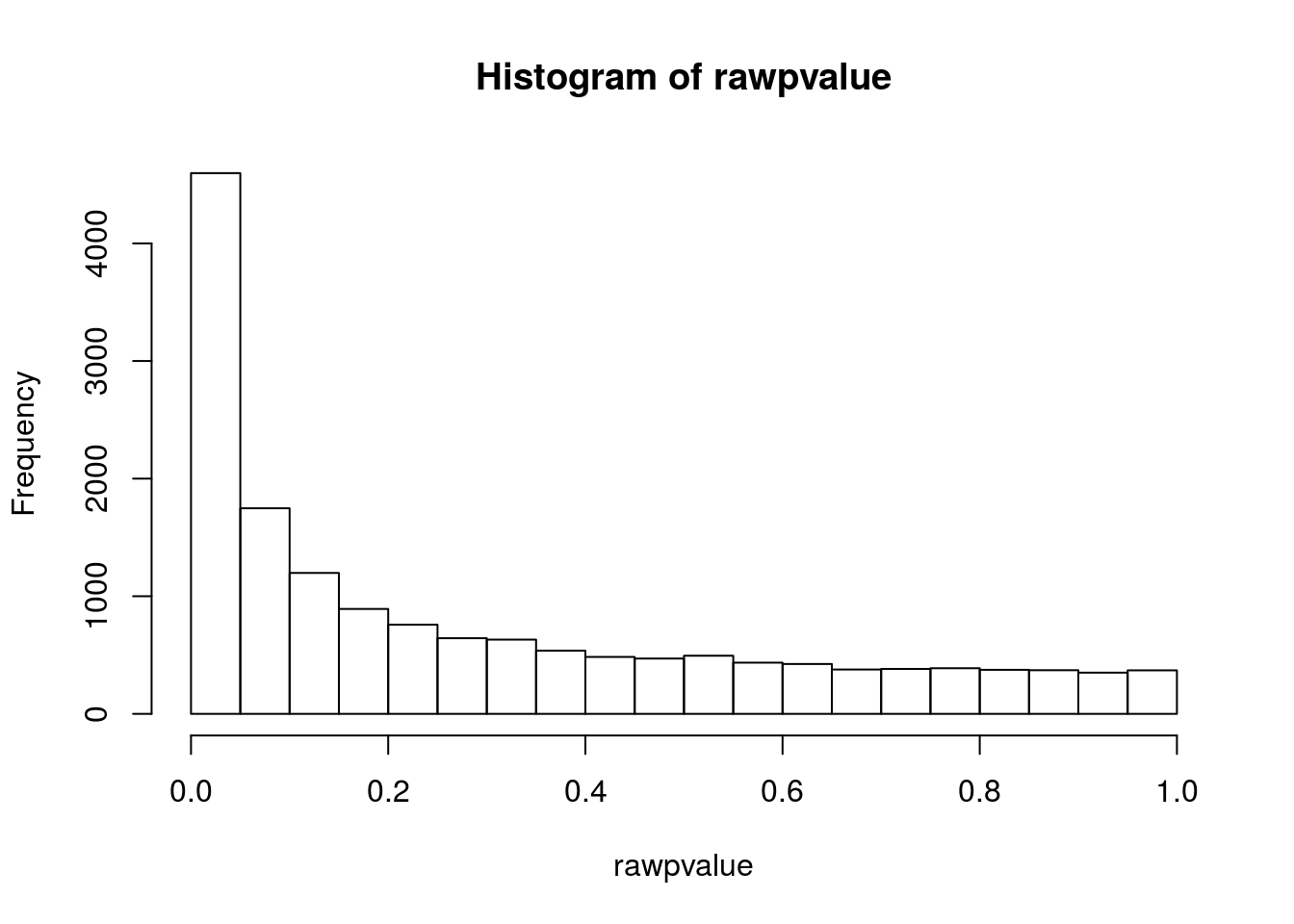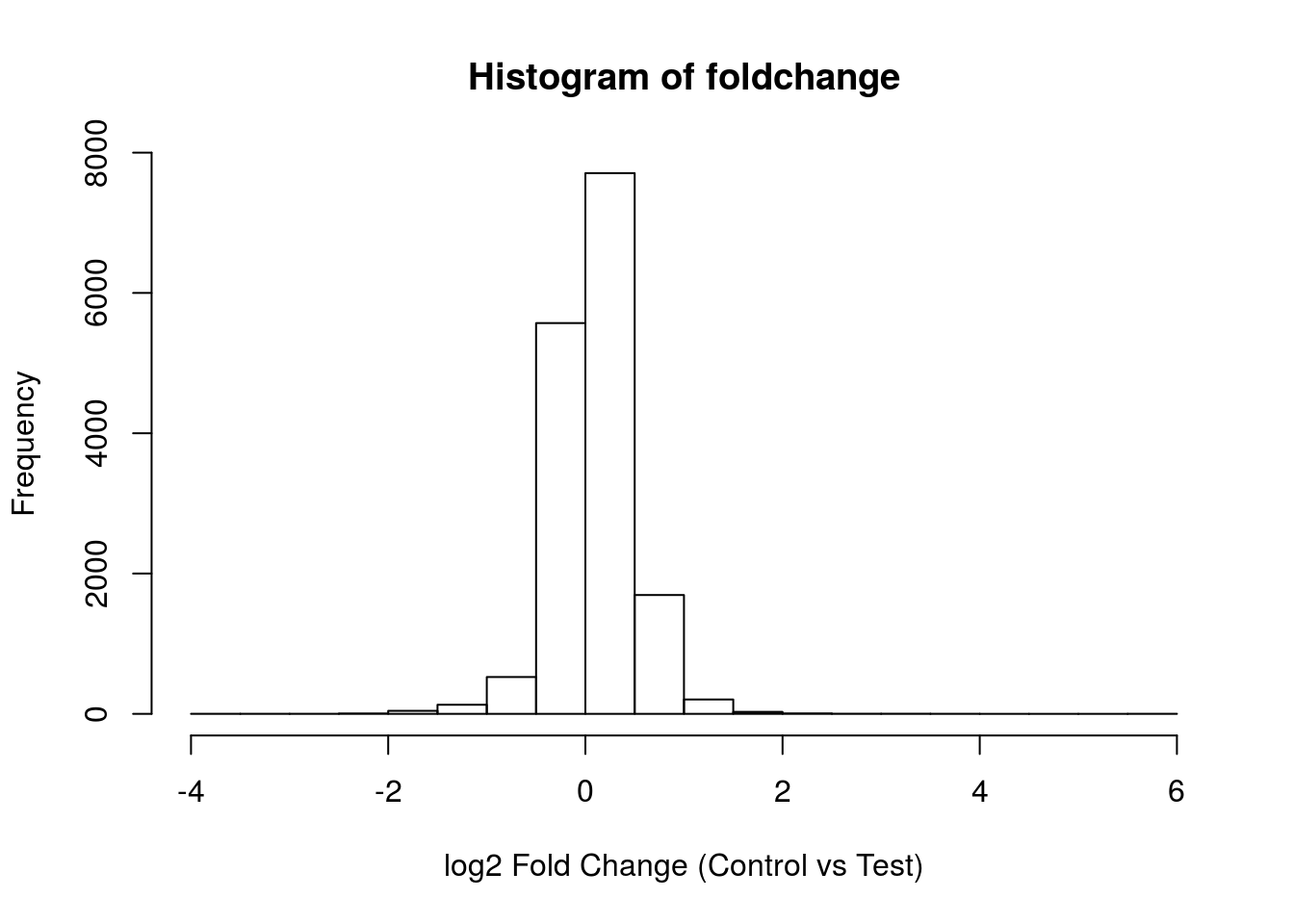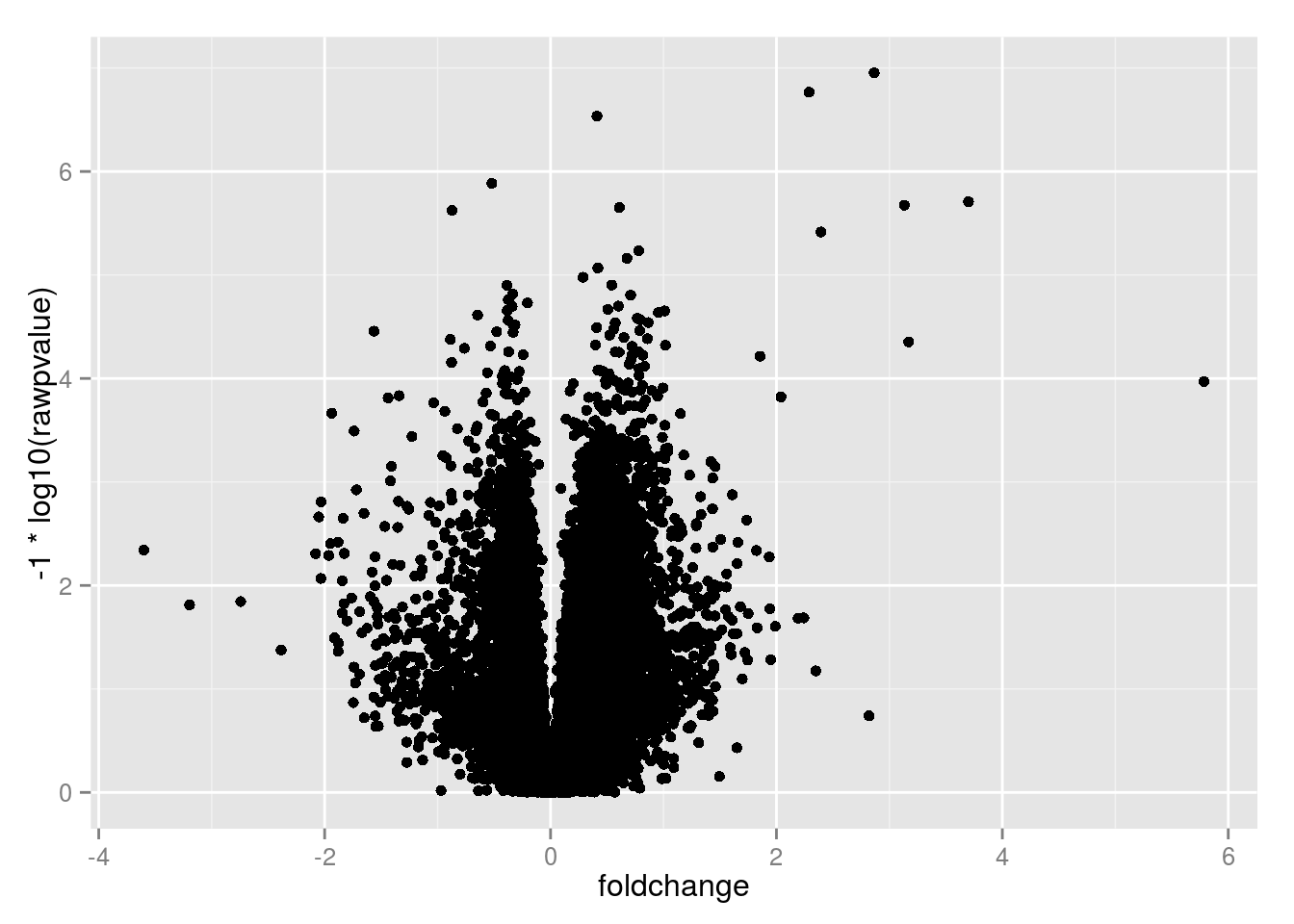Lab #5 Differential expression

1.) Get the GEO rat ketogenic brain data set (rat_KD.txt). rat_KD.txt

rat = read.table("rat_KD.txt", sep = "\t", header = T)
dimnames(rat)[] <- rat[,1]
rat = rat[,-1]

2b.) Set the gene names to row names and remove this first column.

3.) Use the t-test function to calculate the difference per gene between the control diet and ketogenic diet classes. (Hint: use the colnames() function to determine where one class ends and the other begins).

colnames(rat)
##   "control.diet.19300"   "control.diet.19301"   "control.diet.19302"
##   "control.diet.19303"   "control.diet.19304"   "control.diet.19305"
##   "ketogenic.diet.19306" "ketogenic.diet.19307" "ketogenic.diet.19308"
##  "ketogenic.diet.19309" "ketogenic.diet.19310"
t.test(rat[1,1:6], rat[1,7:11])
##
##  Welch Two Sample t-test
##
## data:  rat[1, 1:6] and rat[1, 7:11]
## t = -1.0241, df = 6.1136, p-value = 0.3446
## alternative hypothesis: true difference in means is not equal to 0
## 95 percent confidence interval:
##  -29.69012  12.11468
## sample estimates:
## mean of x mean of y
##  84.63570  93.42342
y <- t.test(rat[2,1:6], rat[2, 7:11])

dim(rat)
##  15923    11
ttestRat <- function(df, grp1, grp2) {
x = df[grp1]
y = df[grp2]
x = as.numeric(x)
y = as.numeric(y)
results = t.test(x, y)
results$p.value } rawpvalue = apply(rat, 1, ttestRat, grp1 = c(1:6), grp2 = c(7:11)) 4.) Plot a histogram of the p-values. hist(rawpvalue)5.) Log2 the data, calculate the mean for each gene per group. Then calculate the fold change between the groups (control vs. ketogenic diet). hint: log2(ratio) ##transform our data into log2 base. rat = log2(rat) #calculate the mean of each gene per control group control = apply(rat[,1:6], 1, mean) #calcuate the mean of each gene per test group test = apply(rat[, 7:11], 1, mean) #confirming that we have a vector of numbers class(control)  ##  "numeric" #confirming we have a vector of numbers class(test) ##  "numeric" #because our data is already log2 transformed, we can take the difference between the means. And this is our log2 Fold Change or log2 Ratio == log2(control / test) foldchange <- control - test  6.) Plot a histogram of the fold change values. hist(foldchange, xlab = "log2 Fold Change (Control vs Test)")7.) Transform the p-value (-1*log(p-value)) and create a volcano plot using ggplot2. results = cbind(foldchange, rawpvalue) results = as.data.frame(results) results$probename <- rownames(results)

library(ggplot2)
volcano = ggplot(data = results, aes(x = foldchange, y = -1*log10(rawpvalue)))
volcano + geom_point()